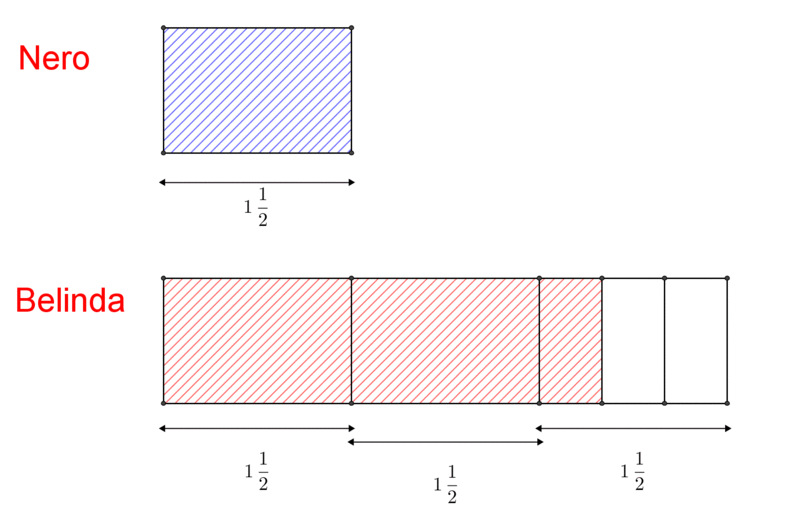Cross Country Training

Alignments to Content Standards: 5.NF.B.4

The members of a cross country team like to continue training on their own during the summer. Nero ran $1\frac{1}{2}$miles one day

1. Lily ran 3 times as far as Nero. How far did Lily run?
2. Jorge ran $\frac{3}{4}$ times as far as Nero. How far did Jorge run?

3. Belinda ran $2\frac{1}{3}$times as far as Nero. How far did Belinda run?

For each question above, show your reasoning with a diagram or a number line. Write a multiplication equation that represents the situation.

IM Commentary

This task was designed to provide students with opportunities to extend their understanding of whole number multiplication to multiplication with fractions.  Students build upon multiplicative comparison that was introduced in fourth grade as they compare two quantities by showing that one quantity is n times farther than the other.

The numbers in the task were intentionally selected to provide students with opportunities to work with fractions greater than 1.  Students multiply a fraction greater than one with a whole number, a fraction smaller than one, and a mixed number.  The variety of fractions may also provide students with an opportunity to analyze numbers and their resulting products, looking for generalizations such as what happens to a fraction when multiplied by a number greater than 1 and a number less than 1. Carefully sequenced diagrams of the three problems can help students see the structure of multiplication as scaling (MP 7); see the last of the three diagrams for each problem.

Students may find it challenging to use a linear model to solve it; they will need to attend carefully to the partition of the number line to get to the denominator of the result. Students may need scaffolding to think about how to subdivide units on the number line.  Partitioning units into halves, then fourths, may be helpful.  Students can then take three from every four sections or simply recognize that 9 out of 12 of these is the $\frac{3}{4}$ they’re looking for, and that they represent eights.

This task was written as part of a collaborative project between Illustrative Mathematics, the Smarter Balanced Digital Library, and the Teaching Channel.

Solution

1. Take three copies of Nero's distance to find Lily's distance. Here are three different diagrams that show Lily ran $4\frac{1}{2}$miles.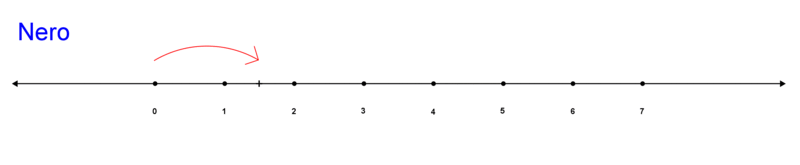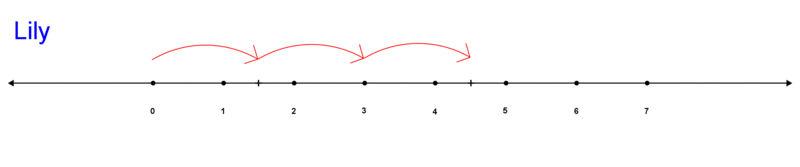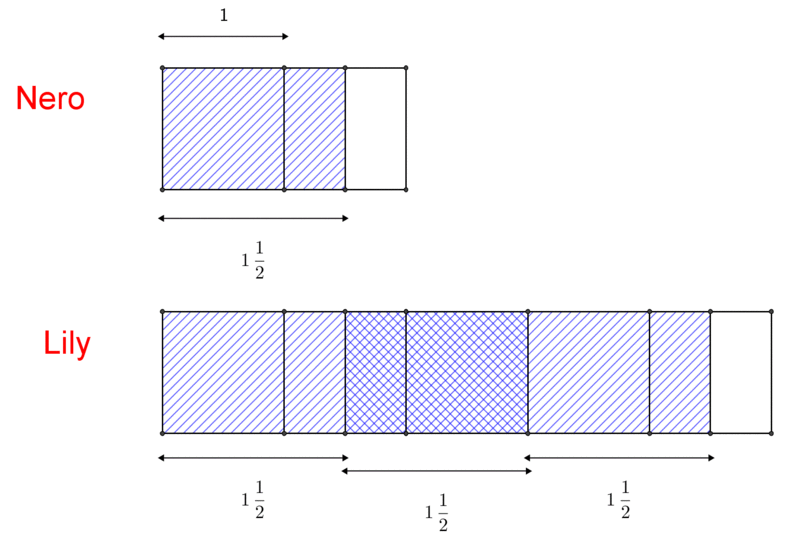1. Partition Nero's distance into four equal pieces and take three of them to find Jorge's distance. Here are three different diagrams that show Jorge ran $1\frac{1}{8}$ miles.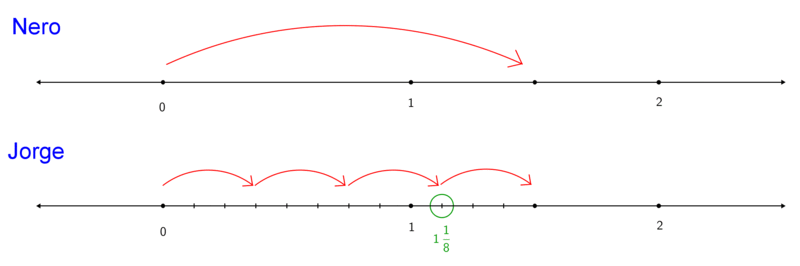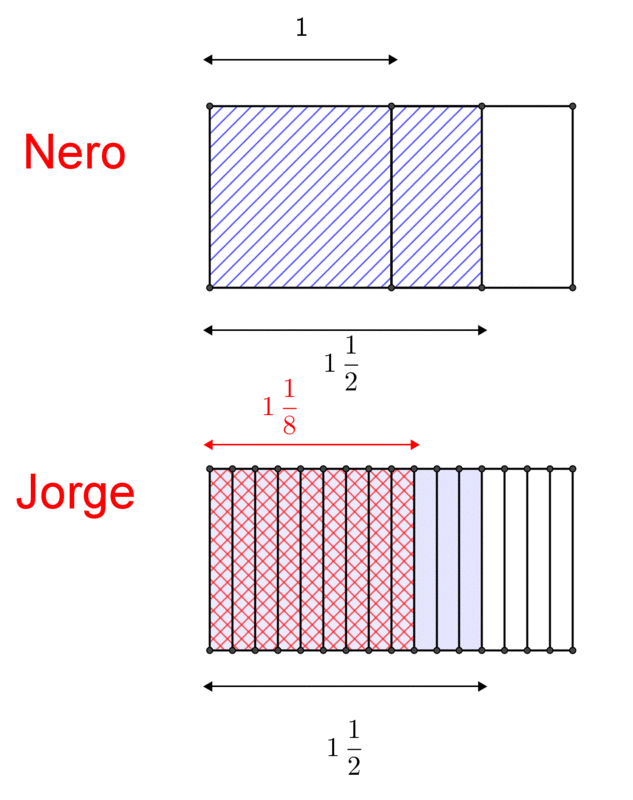1. Take two and a half copies of Nero's distance to find Belinda's distance. Here are three different diagrams that show Belinda ran $3\frac{1}{2}$ miles.